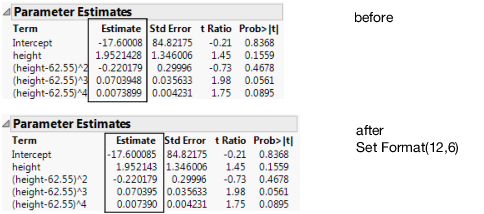Scripting Guide > Display Trees > Navigate JMP Reports > Example of Creating a Report
Publication date: 03/23/2021

## Example of Creating a Report

Here is a script to build a report from start to finish.

1. Open a data table.

`dt = Open( "\$SAMPLE_DATA/Big Class.jmp" );`

2. Launch a Bivariate platform and assign it to the platform reference biv.

`/* a reference to the platform */`
`biv = dt << Bivariate( Y( weight ), X( height ) );`

3. To find out what you can do with the Bivariate platform, search for Bivariate in the Objects list of the Help > Scripting Index. Here are a few options:

`biv << Fit Spline( 1000000 ) << Fit Mean;`
` `

// degree 4, orange curve

`biv << Fit Polynomial( 4, {Line Color( RGBColor( 1, 0.5, 0 ) )});`
`biv << ( Curve << Line Color( red ) ); // first curve is red`

4. Assign rbiv to the report layer:

`rbiv = biv << Report;`

5. Get the frame box to a comfortable size and scroll up to the top to see the graph.

`rbiv[Frame Box( 1 )] << Size Window( 500, 700 );`
`biv << Scroll Window( {0, 0} );`

6. Get to work on the report. First, you need to create a reference, and then see what you can do with it.

`Show Properties( rbiv ); // show the properties of the report`
 Note: The log lists the messages to use with reports. Using Show Properties() is an alternative to the Scripting Index. Close [Boolean] GetOpen [Action] [Scripting Only] SetOpen [Boolean] [Scripting Only] ...

7. Open the Fit Mean node of the outline:

`rbiv["Fit Mean"] << Close( 0 );`

Close() is a Boolean function (true or false), and passing 0 indicates that the outline is not closed.

8. Practice selecting some results (submit each line alone to see its result):

`rbiv["Summary of Fit"] << Select;`
`rbiv["Parameter Estimates"] << Select;`
`rbiv["Analysis of Variance"] << Select;`
`rbiv["Polynomial Fit Degree=4", "Parameter ?", Column Box( "Estimate" )] << Select; // dig down in the outline tree`
`rbiv << Deselect;`

9. Change the format of the Estimate column in the 4-degree polynomial Parameter Estimates report.

`pe = rbiv["Polynomial Fit Degree=4", "Parameter ?"];`
`ests = pe[Number Col Box( "Estimate" )];`
`ests << Set Format( 12, 6 );`

The first argument to Set Format sets the column width by the number of characters to display. The second argument sets how many decimal places are shown in the table.

Figure 11.8 Applying Changes to a ReportNote: You can even get a single number out of the table. For example, get the estimate for the cubic term by adding the following script to the preceding script:`terms = rbiv[Outline Box( "Parameter Estimates" )][String Col Box( "Term" )]; // find the Term column``ests = rbiv[Outline Box( "Parameter Estimates" )][Number Col Box( "Estimate" )]; // find the Estimate column``estimate = .; // in case there is no cubic term``Try(`` For( i = 1, i < 10, i++,`` ` // get(i) goes off the end of the table handled by Try` If( Contains( terms << Get( i ), "^3" ),`` estimate = ests << Get( i );`` Break();`` )`` )``);``Show( estimate );`0.070394822744608

10. Use a For() loop to count down to the row for the term that you want. The second argument to For() is a condition; as long as the condition tests true, looping continues. Here the test is “when the string in the Terms column is not "^3" and we have not reached the tenth row.” As soon as the string does match, looping stops and i’s value is the number for the matching row. You then use i as a subscript to the Get message on the Estimates column.

11. You can also get values from boxes as a matrix, which you can then use for further computations or write to a data table. The following example also shows how to make a data table:

// get the values in the Sum of Squares column as a matrix

`myVector = rbiv[Table Box( 5 )][Number Col Box( "Sum of Squares" )] << Get as Matrix;`
` `

/* create a new column in Big Class.jmp named "Sum of Squares"

insert the values of myVector in the column */

`dt << New Column( "Sum of Squares", Values( myVector ) );`
` `

// put the values of the fifth table box into a new data table

`rbiv[Table Box( 5 )] << Make Data Table( "ANOVA table" );`

12. Now adjust the scales on the axes.

`rbiv[Axis Box( 1 )] << Min( 70 ) << Max( 170 ); // set the Y axis`
`rbiv[Axis Box( 2 )] << Min( 50 ) << Max( 70 );  // set X axis`

13. Copy the graph at the top of the report. Note that you need to select the picture box that contains the graph; selecting just the graph would leave its axes behind.

`rbiv[Picture Box( 1 )] << Copy Picture;`
Want more information? Have questions? Get answers in the JMP User Community (community.jmp.com).